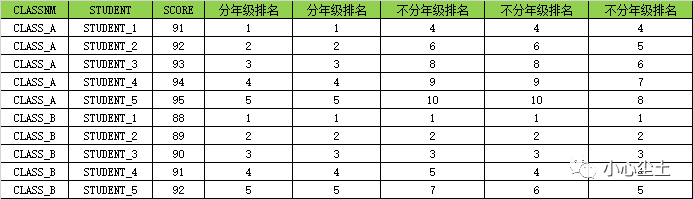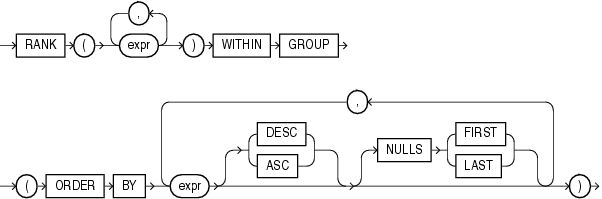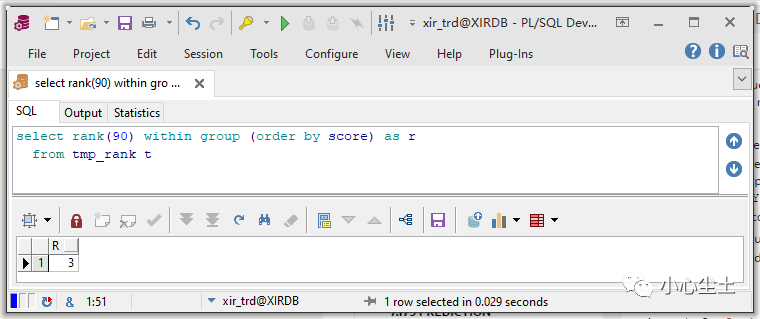create table tmp_rank(
classnm varchar2(10),
student varchar2(10),
score number
);

insert into tmp_rank values('CLASS_A', 'STUDENT_1', 91);
insert into tmp_rank values('CLASS_A', 'STUDENT_2', 92);
insert into tmp_rank values('CLASS_A', 'STUDENT_3', 93);
insert into tmp_rank values('CLASS_A', 'STUDENT_4', 94);
insert into tmp_rank values('CLASS_A', 'STUDENT_5', 95);
insert into tmp_rank values('CLASS_B', 'STUDENT_1', 88);
insert into tmp_rank values('CLASS_B', 'STUDENT_2', 89);
insert into tmp_rank values('CLASS_B', 'STUDENT_3', 90);
insert into tmp_rank values('CLASS_B', 'STUDENT_4', 91);
insert into tmp_rank values('CLASS_B', 'STUDENT_5', 92);
commit;

select t.*,
row_number() over(partition by t.classnm order by t.score) as "分年级排名",
row_number() over(order by t.score) as "不分年级排名",
rank()       over(order by t.score) as "不分年级排名",
dense_rank() over(order by t.score) as "不分年级排名"
from tmp_rank t
order by t.classnm;row_number，传统的排序，结果连续，且与总数一致；

rank，分数相同的情况下，共用一个名次，跳过下一个名次，结果不连续；

dense_rank，分数相同的情况下，共用一个名次，不跳过下一个名次，结果连续；dense_rank，同理。